# Cross Section, Effective

The following article is from The Great Soviet Encyclopedia (1979). It might be outdated or ideologically biased.

## Cross Section, Effective

(or simply, cross section), in physics, a quantity that characterizes the probability of transition of a system of two colliding particles to a certain final state as a result of both elastic and inelastic scattering.

The effective cross section σ is equal to the ratio of the number dN of such transitions per unit time to the density nv of the flux of scattered particles incident on a target, that is, to the number of particles that pass per unit time through unit area perpendicular to the velocity v of the particles (n is the number density of incident particles): σ = dN/nv. Thus, effective cross section has dimensions of an area and consequently is usually measured in cm2.

Different effective cross sections correspond to different types of transitions observed in the scattering of particles. Elastic scattering of particles is characterized by the differential cross section dσ/dΩ, equal to the ratio of the number of particles elastically scattered per unit time per unit solid angle to the flux of incident particles ( is the element of solid angle); it is also characterized by the total cross section σ equal to the integral of the differential cross section over the total solid angle (Ω = 4π steradians). As an example, Figure 1 schematically shows the process of elastic scattering of point “classical” particles by a sphere of radius R0 with an “absolutely rigid” surface. The total effective scattering cross section for this case is equal to the geometric cross section of the sphere: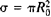.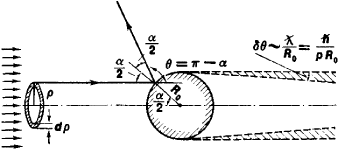Figure 1.

Note to Figure 1. Diagram explaining elastic scattering of a “classical” particle by an “absolutely rigid” sphere. Scattering at an angle θ = π — λ corresponds to an impact parameter ρ = R0 sin (α/2) = R0 cos (θ/2), and the scattering cross section dσ in the solid angle dΩ = 2π sin θdθ is equal to the area of the shaded ring: dσ = 2π ρdρ = (π/2)R02 sin θdθ, that is the differential cross section dσ/dΩ = R02/4, while the total cross section of elastic scattering is equal to the geometric cross section of the sphere: σ = πR02. The cross section is different when the quantum (wave) properties of the particles are taken into account. In the limiting case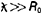, where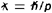is the length of the de Broglie wave of the particle (ρ is the particle’s momentum and ℏ is Planck’s constant), scattering is spherically symmetric, and the total cross section is four times the classical value: σq = 4πR02. At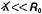, scattering at finite angles (θ ≠ O) is similar to the classical case; however, at very small angles,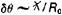, wave “diffraction” scattering occurs with cross section πR02: thus the total cross section with regard to diffraction is twice the classical value: σ = 2πR02.

In the presence of inelastic processes, the total cross section is the sum of the effective cross sections of elastic and inelastic processes. The cross section for individual types (channels) of inelastic reactions is introduced for more detailed characterization of scattering. Partial cross sections describing the probability that a certain particle or group of particles will show up in a given collision are important for multiple processes.

If interaction between the colliding particles is strong and decreases rapidly with distance, the effective cross section as a rule is equal in order of magnitude to the square of the radius of action of the forces or to the geometric cross section of the system (see Figure 1); however, as a result of specific quantum-mechanical phenomena, the effective cross sections may differ considerably from these values (for example, in cases of resonance scattering and the Ramsauer effect).

Experimental measurements of effective scattering cross sections provide information on the structure of the colliding particles. For example, measurements of the cross section of the elastic scattering of alpha particles by atoms led to the discovery of the atomic nucleus, and measurements of the cross section of the elastic scattering of electrons by protons and neutrons (nucleons) made possible the determination of the radii of nucleons and the distribution of the electric charge and magnetic moment within nucleons (the form factors). The concept of effective cross section is also used in statistical physics for setting up kinetic questions.

S. S. GERSHTEIN